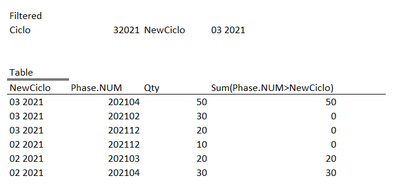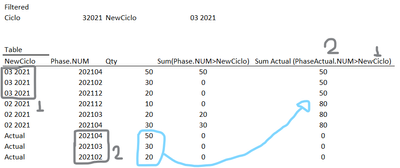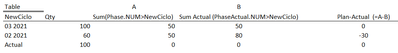# QlikView App Dev

Discussion Board for collaboration related to QlikView App Development.

Announcements
Action-Packed Learning Awaits! QlikWorld 2023. April 17 - 20 in Las Vegas: REGISTER NOW
cancel
Showing results for
Did you mean:Contributor II

## Set Analysis Greater than variable from row

Hello Everyone,

What I am trying to achieve seems very easy, but I am facing some troubles.

I Have basically 3 dimensions:

Phase.NUM= Is a number (yyyymm),
Ciclo = Cycle (number) (myyyy),

NewCiclo = Cycle as text (mm yyyy),

I am trying to get a table with the Sum of a "Total" only if my "Phase.NUM" is greater than my "NewCiclo", which need to be adjusted to the same format as Phase. Additionally, I have a filter applied on this page for Cycle Field that should be ignored for this table.

I could achieve partially with the code bellow, however, its not dynamically calculating based on every row of my table.

The part {">\$(=Right(NewCiclo,4)&Left(NewCiclo,2))"} is bringing a fixed value following the filter selected not the "NewCiclo" value from each row.

Code:

SUM( {1}
aggr({1}

sum({\$<[Phase.NUM]={">\$(=Right(NewCiclo,4)&Left(NewCiclo,2))"}, Ciclo >}Total)

material, Ciclo

)
)

The expected result should be something like this:Aggregating without "Phase.NUM" should get this:1 Solution

Accepted SolutionsMVP

You cannot create a set using row values. The set is calculated once for the chart. To do row by row processing you must use if(). In your case:

sum({\$<[Ciclo= >}if([Phase.NUM] > Right(NewCiclo,4)& Left(NewCiclo,2), Total))

3 RepliesMVP

You cannot create a set using row values. The set is calculated once for the chart. To do row by row processing you must use if(). In your case:

sum({\$<[Ciclo= >}if([Phase.NUM] > Right(NewCiclo,4)& Left(NewCiclo,2), Total))Contributor II
Author

Thanks a lot, it worked!!!

Another tricky question... I need to do the same for the same row, based on a value from other row just like the table below.

I tried analogous with the previous solution, but no success.

(sum({\$<Ciclo={'Actual'}>} if([Phase.NUM] >= Right(NewCiclo,4)& Left(NewCiclo,2), Total) + Sum({1} 0)))

What is getting complex, I guess, is that for this code the Phase.NUM parameter that I need is from rows which "NewCiclo = Actual" and the NewCiclo parameter is from the current row, I'm mixing up the things a little bit.The idea afterwards is to achieve this:MVP

You'll need to use the TOTAL modifier to reference rows "outside your dimension"

https://help.qlik.com/en-US/sense/February2021/Subsystems/Hub/Content/Sense_Hub/ChartFunctions/Basic...

-RobCommunity Browser